Related Articles

# Perl | Loops (for, foreach, while, do…while, until, Nested loops)

• Last Updated : 16 Jun, 2021

Looping in programming languages is a feature which facilitates the execution of a set of instructions or functions repeatedly while some condition evaluates to true. Loops make the programmers task simpler. Perl provides the different types of loop to handle the condition based situation in the program. The loops in Perl are :

for Loop

“for” loop provides a concise way of writing the loop structure. Unlike a while loop, a for statement consumes the initialization, condition and increment/decrement in one line thereby providing a shorter, easy to debug structure of looping.
Syntax:

```for (init statement; condition; increment/decrement )
{
# Code to be Executed
}```

Flow Chart: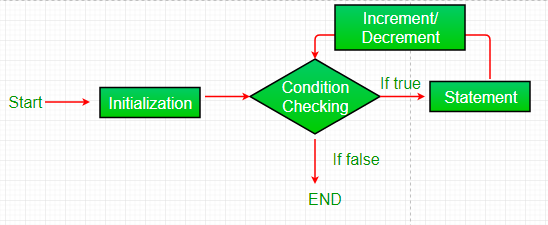A for loop works on a predefined flow of control. The flow of control can be determined by the following :

• init statement: This is the first statement which is executed. In this step, we initialize a variable which controls the loop.
• condition: In this step, the given condition is evaluated and the for loop runs if it is True. It is also an Entry Control Loop as the condition is checked prior to the execution of the loop statements.
• Statement execution: Once the condition is evaluated to true, the statements in the loop body are executed.
• increment/decrement: The loop control variable is changed here (incremented or decremented) for updating the variable for next iteration.
• Loop termination: When the condition becomes false, the loop terminates marking the end of its life cycle.

Example :

## Perl

 `# Perl program to illustrate``# the for loop` `# for loop``for` `(``\$count` `= 1 ; ``\$count` `<= 3 ; ``\$count``++)``{``    ``print` `"GeeksForGeeks\n"``}`

Output:

```GeeksForGeeks
GeeksForGeeks
GeeksForGeeks```

foreach Loop

A foreach loop is used to iterate over a list and the variable holds the value of the elements of the list one at a time. It is majorly used when we have a set of data in a list and we want to iterate over the elements of the list instead of iterating over its range. The process of iteration of each element is done automatically by the loop.
Syntax:

```foreach variable
{
# Code to be Executed
}```

Flow Chart: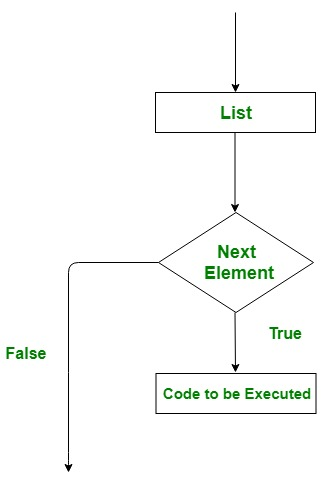Example:

## Perl

 `# Perl program to illustrate``# the foreach loop` `# Array``@data` `= (``'GEEKS'``, ``'FOR'``, ``'GEEKS'``);` `# foreach loop``foreach` `\$word` `(``@data``)``{``    ``print` `\$word``}`

Output:

`GEEKSFORGEEKS`

while Loop

A while loop generally takes an expression in parenthesis. If the expression is True then the code within the body of while loop is executed. A while loop is used when we don’t know the number of times we want the loop to be executed however we know the termination condition of the loop. It is also known as a entry controlled loop as the condition is checked before executing the loop. The while loop can be thought of as a repeating if statement.
Syntax :

```while (condition)
{
# Code to be executed
}```

Flow Chart: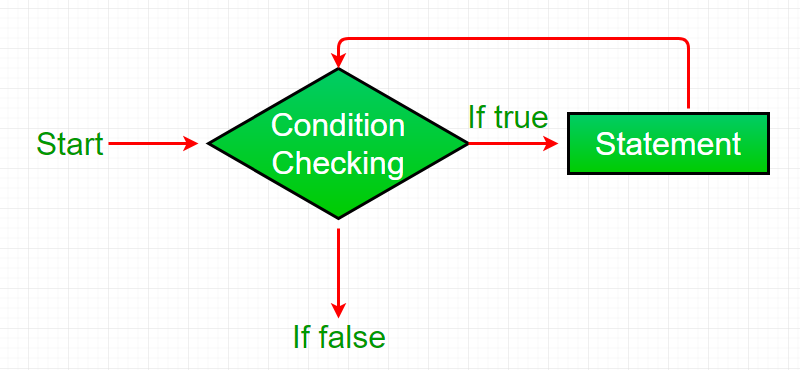Example :

## Perl

 `# Perl program to illustrate``# the while loop` `# while loop``\$count` `= 3;``while` `(``\$count` `>= 0)``{``    ``\$count` `= ``\$count` `- 1;``    ``print` `"GeeksForGeeks\n"``;``}`

Output:

```GeeksForGeeks
GeeksForGeeks
GeeksForGeeks
GeeksForGeeks```

Infinite While Loop: While loop can execute infinite times which means there is no terminating condition for this loop. In other words, we can say there are some conditions which always remain true, which causes while loop to execute infinite times or we can say it never terminates.

• Example: Below program will print the specified statement infinite time and also give the runtime error as Output Limit Exceeded on online IDE

## Perl

 `# Perl program to illustrate``# the infinite while loop` `# infinite while loop``# containing condition 1``# which is always true``while``(1)``{``    ``print` `"Infinite While Loop\n"``;``}`
• Output:

```Infinite While Loop
Infinite While Loop
Infinite While Loop
Infinite While Loop
.
.
.
.```

do…. while loop

A do..while loop is almost same as a while loop. The only difference is that do..while loop runs at least one time. The condition is checked after the first execution. A do..while loop is used when we want the loop to run at least one time. It is also known as exit controlled loop as the condition is checked after executing the loop.
Syntax:

```do {

# statements to be Executed

} while(condition);```

Flow Chart: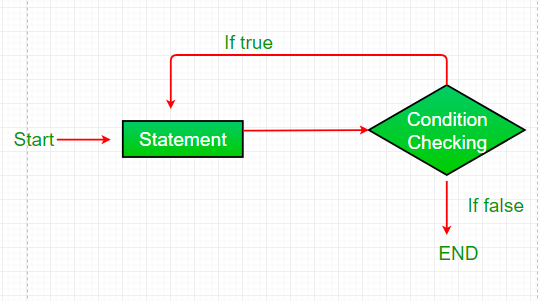Example :

## Perl

 `# Perl program to illustrate``# do..while Loop` `\$a` `= 10;` `# do..While loop``do` `{` `    ``print` `"\$a "``;``    ``\$a` `= ``\$a` `- 1;``} ``while` `(``\$a` `> 0);`

Output:

`10 9 8 7 6 5 4 3 2 1`

until loop

until loop is the opposite of while loop. It takes a condition in the parenthesis and it only runs until the condition is false. Basically, it repeats an instruction or set of instruction until the condition is FALSE. It is also entry controller loop i.e. first the condition is checked then set of instructions inside a block is executed.
Syntax:

```until (condition)
{
# Statements to be executed
}```

Flow Chart: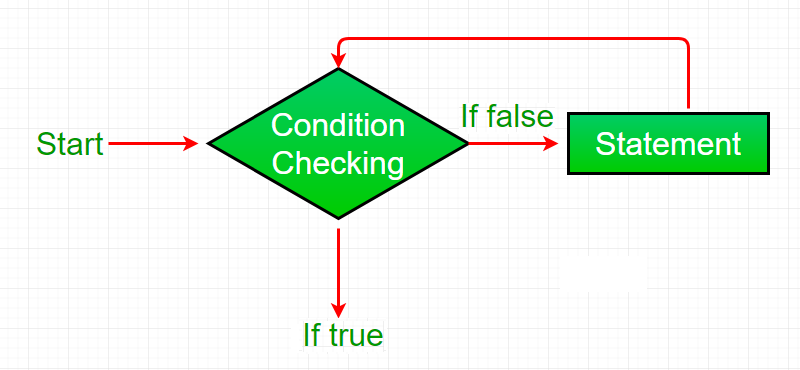Example :

## Perl

 `# Perl program to illustrate until Loop` `\$a` `= 10;` `# until loop``until` `(``\$a` `< 1)``{``    ``print` `"\$a "``;``    ``\$a` `= ``\$a` `- 1;``}`

Output:

`10 9 8 7 6 5 4 3 2 1`

Nested Loops

A nested loop is a loop inside a loop. Nested loops are also supported by Perl Programming. And all above-discussed loops can be nested.
Syntax for different nested loops in Perl:

• Nested for loop

```for (init statement; condition; increment/decrement )
{
for (init statement; condition; increment/decrement )
{
# Code to be Executed
}
}```
•
• Nested foreach loop

```foreach variable_1 (@array_1) {

foreach variable_2 (@array_2)
{

# Code to be Executed
}
}```
•
• Nested while loop

```while (condition)
{
while (condition)
{
# Code to be Executed
}
}```
•
• Nested do..while loop

```do{
do{

# Code to be Executed

}while(condition);

}while(condition);```
• Nested until loop

```until (condition) {

until (condition)
{
# Code to be Executed
}
}```
•

Example :

## Perl

 `# Perl program to illustrate``# nested while Loop` `\$a` `= 5;``\$b` `= 0;` `# outer while loop``while` `(``\$a` `< 7)``{``   ``\$b` `= 0;``   ` `   ``# inner while loop``   ``while` `( ``\$b` `<7 )``   ``{``      ``print` `"value of a = \$a, b = \$b\n"``;``      ``\$b` `= ``\$b` `+ 1;``   ``}``   ` `   ``\$a` `= ``\$a` `+ 1;``   ``print` `"Value of a = \$a\n\n"``;``}`

Output:

```value of a = 5, b = 0
value of a = 5, b = 1
value of a = 5, b = 2
value of a = 5, b = 3
value of a = 5, b = 4
value of a = 5, b = 5
value of a = 5, b = 6
Value of a = 6

value of a = 6, b = 0
value of a = 6, b = 1
value of a = 6, b = 2
value of a = 6, b = 3
value of a = 6, b = 4
value of a = 6, b = 5
value of a = 6, b = 6
Value of a = 7```

My Personal Notes arrow_drop_up